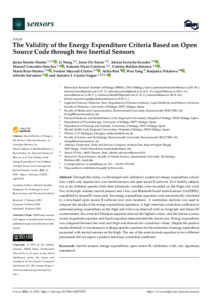# The Validity of the Energy Expenditure Criteria Based on Open Source Code through two Inertial Sensors.

Martín-Martín, J., Wang, L., De-Torres, I., Escriche-Escuder, A., González-Sánchez, M., Muro-Culebras, A., Roldán-Jiménez, C., Ruiz-Muñoz, M., Mayoral-Cleries, F., Biró, A., Tang, W., Nikolova, B., Salvatore, A. and Cuesta-Vargas, A.I., 2022. The Validity of the Energy Expenditure Criteria Based on Open Source Code through two Inertial Sensors. Sensors, 22 (7), 2552.

Full text available as:Preview
PDF (OPEN ACCESS ARTICLE)
sensors-22-02552-v2.pdf - Published Version

314kB

## Abstract

Through this study, we developed and validated a system for energy expenditure calcula-tion, which only requires low-cost inertial sensors and open source R software. Five healthy subjects ran at ten different speeds while their kinematic variables were recorded on the thigh and wrist. Two ActiGraph wireless inertial sensors and a low-cost Bluetooth-based inertial sensor (Lis2DH12), assembled by SensorID, were used. Ten energy expenditure equations were automatically calculated in a developed open source R software (our own creation). A correlation analysis was used to compare the results of the energy expenditure equations. A high interclass correlation coefficient of estimated energy expenditure on the thigh and wrist was observed with an Actigraph and Sensor ID accelerometer; the corrected Freedson equation showed the highest values, and the Santos-Lozano vector magnitude equation and Sasaki equation demonstrated the lowest one. Energy expenditure was compared between the wrist and thigh and showed low correlation values. Despite the positive results obtained, it was necessary to design specific equations for the estimation of energy expenditure measured with inertial sensors on the thigh. The use of the same formula equation in two different placements did not report a positive interclass correlation coefficient.

Item Type: Article 1424-8220 This article belongs to the Special Issue Wearable Sensors for Gait and Falls Monitoring inertial sensors; energy expenditure; open source R; validation; walk; run; equations; assessment Faculty of Science & Technology 36866 Symplectic RT2 25 Apr 2022 09:59 25 Apr 2022 09:59